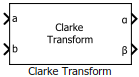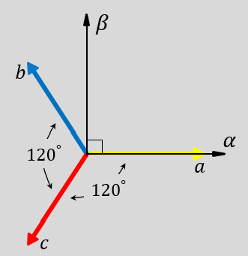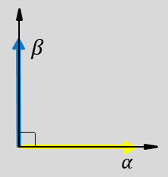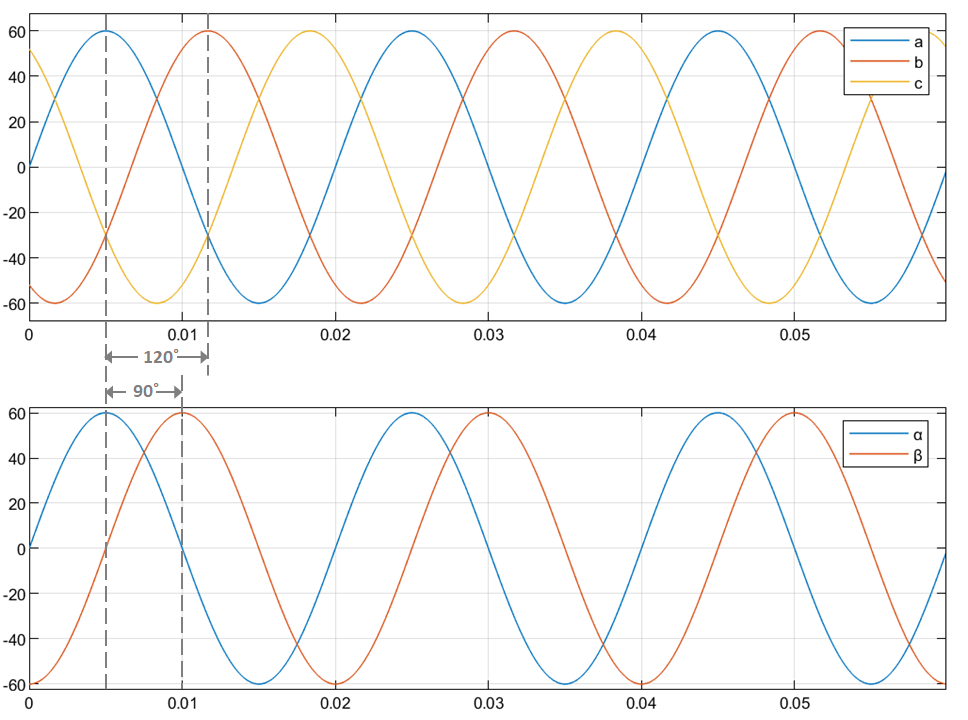# Clarke Transform

Implement ab to αβ transformation

Since R2020a

•Libraries:
Motor Control Blockset / Controls / Math Transforms
Motor Control Blockset HDL Support / Controls / Math Transforms

## Description

The Clarke Transform block computes the Clarke transformation of balanced three-phase components in the abc reference frame and outputs the balanced two-phase orthogonal components in the stationary αβ reference frame. Alternatively, the block can compute Clarke transformation of three-phase components a, b, and c and output the components α, β, and `0`. For a balanced system, the zero component is equal to zero. Use the Number of inputs parameter to use either two or three inputs.

When using two-input configuration, the block accepts two signals out of the three phases (abc), automatically calculates the third signal, and outputs the corresponding components in the αβ reference frame.

For example, the block accepts either a and b input values or the multiplexed input value abc where the phase-a axis aligns with the α-axis.

• This figure shows the direction of the magnetic axes of the stator windings in the abc reference frame and the stationary αβ reference frame.• This figure shows the equivalent α and β components in the stationary αβ reference frame.• The time-response of the individual components of equivalent balanced abc and αβ systems.### Equations

The following equation describes the Clarke transform computation:

For balanced systems like motors, the zero sequence component calculation is always zero. For example, the currents of the motor can be represented as,

`${i}_{a}+{i}_{b}+{i}_{c}=0$`

Therefore, you can use only two current sensors in three-phase motor drives, where you can calculate the third phase as,

`${i}_{c}=-\left({i}_{a}+{i}_{b}\right)$`

By using these equations, the block implements the Clarke transform as,

where:

• ${f}_{a}$, ${f}_{b}$, and ${f}_{c}$ are the balanced three-phase components in the abc reference frame.

• ${f}_{\alpha }$ and ${f}_{\beta }$ are the balanced two-phase orthogonal components in the stationary αβ reference frame.

• ${f}_{0}$ is the zero component in the stationary αβ reference frame.

## Ports

### Input

expand all

Component of the three-phase system in the abc reference frame.

#### Dependencies

To enable this port, set the Number of inputs parameter to `Two inputs`.

Data Types: `single` | `double` | `fixed point`

Component of the three-phase system in the abc reference frame.

#### Dependencies

To enable this port, set the Number of inputs parameter to `Two inputs`.

Data Types: `single` | `double` | `fixed point`

Multiplexed phase components a,b, and c of the three-phase system in the abc reference frame.

#### Dependencies

To enable this port, set the Number of inputs parameter to `Three inputs`.

Data Types: `single` | `double` | `fixed point`

### Output

expand all

Alpha-axis component, α, in the stationary αβ reference frame.

#### Dependencies

To enable this port, set the Number of inputs parameter to `Two inputs`.

Data Types: `single` | `double` | `fixed point`

Beta-axis component, β, in the stationary αβ reference frame.

#### Dependencies

To enable this port, set the Number of inputs parameter to `Two inputs`.

Data Types: `single` | `double` | `fixed point`

Multiplexed alpha-axis component, α and beta-axis component, β and `0` component, in the stationary αβ reference frame.

#### Dependencies

To enable this port, set the Number of inputs parameter to `Three inputs`.

Data Types: `single` | `double` | `fixed point`

## Parameters

expand all

Select the number of inputs that you can specify:

• `Two inputs` — Configure the block to accept two separate input signals a and b. The block generates two separate output signals α and β.

• `Three inputs` — Configure the block to accept a multiplexed input containing a,b, and c signals. The block generates a multiplexed output containing α, β, and 0 signals.

To enable the power invariance property in the block output, select this parameter. To disable power invariance (enable amplitude invariance) in the block output, clear this parameter.

## Version History

Introduced in R2020a